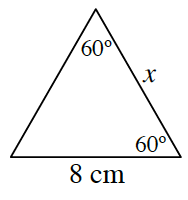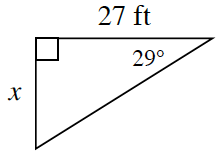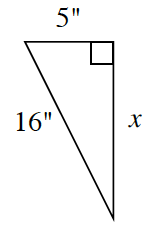### Home > CCG > Chapter 6 > Lesson 6.1.1 > Problem6-7

6-7.

For each of the triangles below, find $x$.

1.Find the measure of the unknown angle.
What does this measure tell you about the lengths of the sides?

1.Which trigonometric ratio can you use to find the unknown side?

$\tan29º=\frac{x}{27}$

$27\tan29º=x$

$x\approx14.9663$ feet

1.Use the Pythagorean Theorem to find the length of the unknown side.